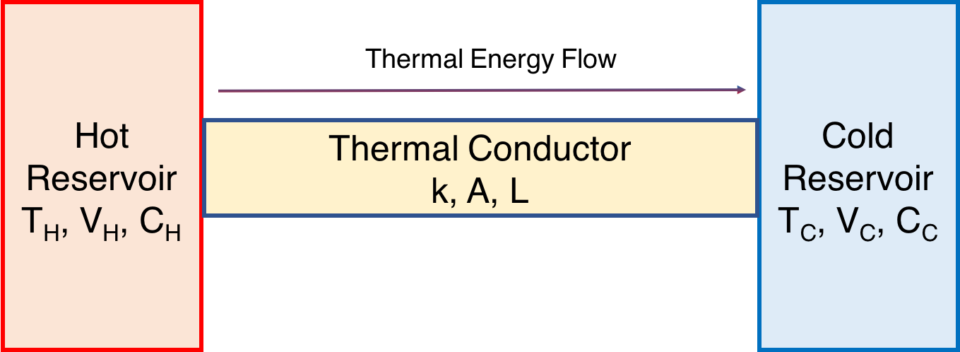# Fourier’s Law of Heat Conduction

First published on April 30, 2021. Last updated on May 23, 2021.

Fourier’s Law of Conduction concerns thermal energy flowing from a warmer body of to a cooler body through a thermal conductor. We call the bodies reservoirs. Flowing thermal energy is often called heat.

Let us consider a thermal conductor operating upon in exhaustible reservoirs. The hot reservoir could be a flame or a large tank of hot water. The cold reservoir could be radiator fin or cooling pond. The hot reservoir has thermal energy to spare, and the cold reservoir wants some of that energy.  The rate of thermal energy transfer is known as Q. The “hot” reservoir has a temperature of TH, and the “cold” reservoir has a temperature of TC. The conductor is of area (cross section) A, and of length L.If you are a knowledgeable engineer or scientist, you might point out that such thermal reservoirs have many important characteristics such as volume and specific heat capacity, but here we will simplify matters and assume that those are identical for both reservoirs and that there are no phase changes. Only their temperatures differ, the hot reservoir being at 300 and 500 “degrees” Kelvin (actually a unit just called Kelvin (K) with is similar to degrees). So the temperature difference is 200 K.

The flow of thermal energy is:

$$\Large{\frac{dQ}{dt}}=kA\frac{T_H – T_C}{L}$$.

The quantity of heat energy lost by the warmer body is identical to the quantity of heat energy gained by the cooler body. This must be true according to the First Law of Thermodynamics in order for energy to be conserved. That is, energy neither gained nor lost for the overall system. (Our conductor is well insulated except where it contacts the reservoirs).

Notice how the higher the temperature difference, the greater will be the flow of thermal energy. A large difference shall result in a large flow. A small difference will result in a small flow.

Note: Some thermodynamics textbooks or chapters begin with a discussion of Newton’s Law of Cooling. Fourier’s Law is a broader principle that encompasses Newton’s Law of Cooling.